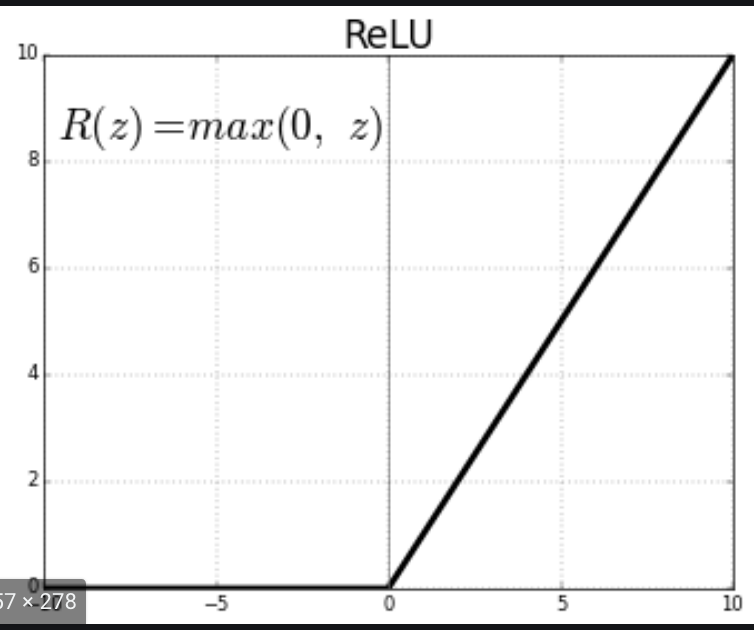# How is the performance of a model affected by adding a ReLU to fully connected layers?

How significant is adding a ReLU to fully connected (FC) layers? Is it necessary, or how is the performance of a model affected by adding ReLU to FC layers?

ReLU is piecewise linear function that outputs the received input directly if it's positive, or outputs a zero. i.e., $$max(0, x)$$### How significant is adding relu to full connected layers?

ReLU, being an activation function, will determine what the output of the nodes in your FCs are. Since it's a non-linear function, one significance is it will allow the nodes in your model to learn complex mappings between the inputs and the outputs. Compared to using a linear function, it will allow back-propagation since it has a derivative, allowing the neural network to have the advantage of stacking of multiple FC layers.

### Is it necessary?

ReLU (and non-linear activation functions in general) will introduce non-linear properties in a neural network that enables it to learn more complex arbitrary structures in the inputs. Without activation functions between the layers, your neural network will simply be a linear function, regardless of the number of layers it has. Why? A linear function + linear function gives a linear function. Additionally, see this answer.

### How is the performance affected by adding ReLU?

Compared to no activation function at all, it will be slower to train but will only behave like a linear regression model. So ReLU will increase the power of the model by making it non-linear. However, compared to other non-linear activation functions like tanH, ReLU will speed up training as (1) its computation step is cheaper i.e., $$0.0$$ or $$x$$ without additional operations (2) its gradient just depends on the sign of the input $$x$$. See this answer